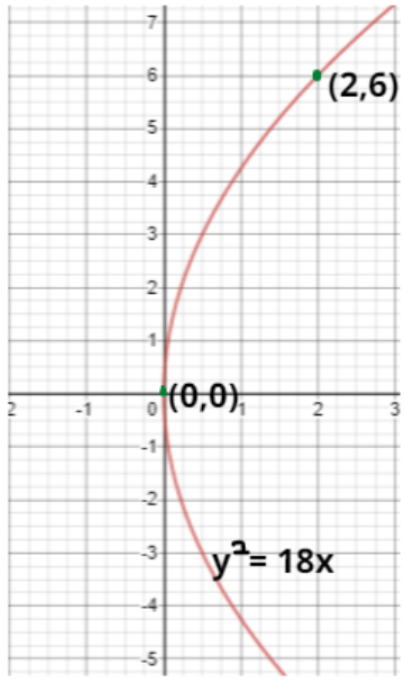QuestionAnswers

# For what point of the parabola the ${y^2} = 18x$ ordinate equal to three times the abscissa?Verified
161.1k+ views
Hint: Substitute the relation between abscissa and ordinate into the given parabola equation.As, we know that we are given with the parabola
${y^2} = 18x$ …………………………………………………….. (1)
We know that abscissa is the x-coordinate and ordinate is the y-coordinate.
Let the abscissa and ordinate of the point on parabola be ${x_1}$ and ${y_1}$ respectively.
So, according to the given condition. Ordinate of the point will be equal to three times the abscissa i.e. ${y_1} = 3{x_1}$.
So, as the points lie on the parabola the coordinates of the points must satisfy the equation of parabola.
Now substituting the coordinates of the points in equation (1) we get,
$\Rightarrow {y_1}^2 = 18{x_1} \\ \Rightarrow {\left( {3{x_1}} \right)^2} = 18{x_1} \\ \Rightarrow {9x_1}\left( {{x_1} - 2} \right) = 0 \\ \Rightarrow {x_1} = 0,2 \\$
Hence,
For ${x_1} = 0 \Rightarrow {y_1} = 0$, Point$\left( {0,0} \right)$
For ${x_1} = 2 \Rightarrow {y_1} = 6$, Point$\left( {2,6} \right)$

Note: Whenever we come across these types of problems then we have to remember that if a point lies on the curve then its coordinates must satisfy the equation of the curve.